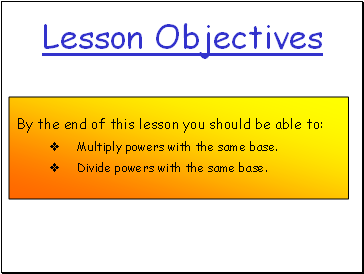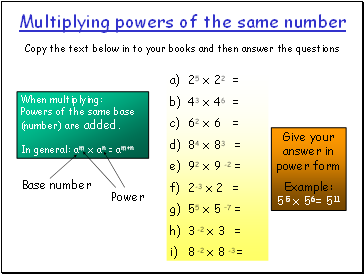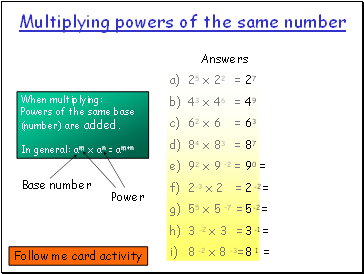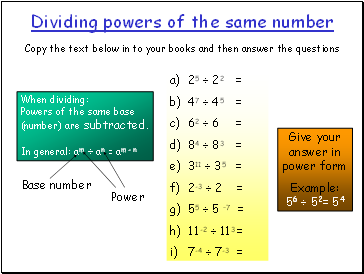# Multiplying & Dividing PowersPage 1

#### WATCH ALL SLIDES

Slide 1## Lesson Objectives

By the end of this lesson you should be able to:

Multiply powers with the same base.

Divide powers with the same base.

Slide 2## Multiplying powers of the same number

Copy the text below in to your books and then answer the questions

When multiplying:

Powers of the same base (number) are added.

In general: am x an = am+n

25 x 22 =

43 x 46 =

62 x 6 =

84 x 83 =

92 x 9 -2 =

2-3 x 2 =

55 x 5 –7 =

3 -2 x 3 =

8 -2 x 8 -3 =

Example:

55 x 56= 511

Base number

Power

Slide 3Multiplying powers of the same number

When multiplying:

Powers of the same base (number) are added.

In general: am x an = am+n

25 x 22 =

43 x 46 =

62 x 6 =

84 x 83 =

92 x 9 –2 =

2-3 x 2 =

55 x 5 –7 =

3 -2 x 3 =

8 -2 x 8 -3 =

27

49

63

87

90 =

2 -2 =

5 -2 =

3 -1 =

8 1 =

Base number

Power

Slide 4## Dividing powers of the same number

Copy the text below in to your books and then answer the questions

When dividing:

Powers of the same base (number) are subtracted.

In general: am ÷ an = am - n

25 ÷ 22 =

47 ÷ 45 =

62 ÷ 6 =

84 ÷ 83 =

311 ÷ 35 =

2-3 ÷ 2 =

55 ÷ 5 –7 =

11 -2 ÷ 113 =

7-4 ÷ 7-3 =

Example:

56 ÷ 52= 54

Base number

Power

Slide 5When dividing:

Powers of the same base (number) are subtracted.

In general: am ÷ an = am - n

25 ÷ 22 =

47 ÷ 45 =

62 ÷ 6 =

84 ÷ 83 =

311 ÷ 35 =

2-3 ÷ 2 =

55 ÷ 5 –7 =

11 -2 ÷ 113 =

7-4 ÷ 7-3 =

Dividing powers of the same number

23

42

61 =

81 =

36

2 -4

512

11 -5

7-1 =

Base number

Power

Now try these:

2 x 25

21

35 x 3-2

32

5-3 x 54

5-2

42 x 46

4-7

Extra Questions